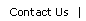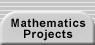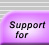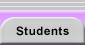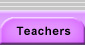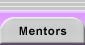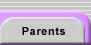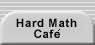# Proof

Proof is central to mathematics inquiry. It is at the heart of what we mean when we claim to know that a mathematical result is true. Proving theorems is the exciting, frustrating, and unavoidable challenge faced by all mathematics researchers. Proofs are also a means of gaining insight into a mathematical question.

Consult the discussions and exercises in the proof section of the teacher handbook.

For specific proof techniques, see:

Conditional StatementsTranslations of mathematical formulas for web display were created by tex4ht. © Copyright 2003 Education Development Center, Inc. (EDC)